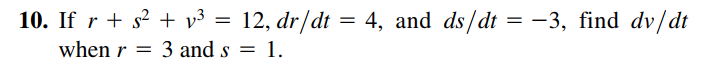# 10. If r + s? + v³ = 12, dr/dt = 4, and ds/dt = -3, find dv/dt when r = 3 and s = 1.

Question

Can i get help step by step pleasehelp_outlineImage Transcriptionclose10. If r + s? + v³ = 12, dr/dt = 4, and ds/dt = -3, find dv/dt when r = 3 and s = 1. fullscreen